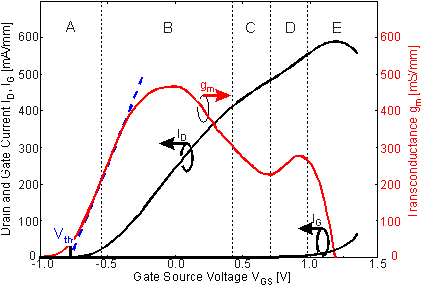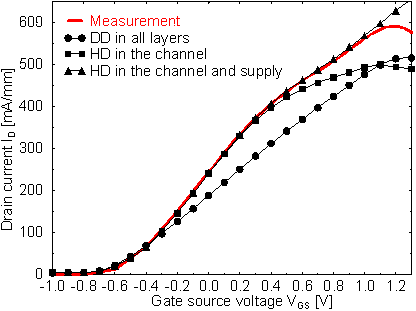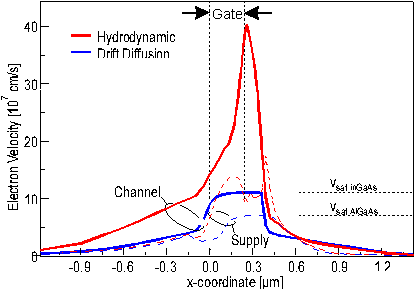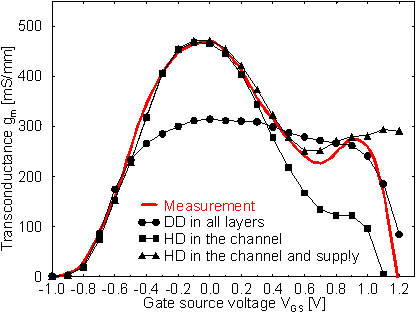Next: 5.3 Determination of the Parameter Set for the Simulation Up: 5 Simulation Previous: 5.1 Composition of the Simulated Device Geometry

5.2 Determination of the Transport Models

In the following section different combinations of transport models will be investigated. Some models can lead to significant increase in computation time or to convergence problems, for example the combination of the hydrodynamic transport and the thermionic field emission interface model. Therefore it is necessary to find the optimum simulation setup for a given task.

In HEMTs current transport in three main layers under the gate has to be considered, namely in the barrier layer below the channel, in the channel itself, and in the upper barrier layer. The impact of employing different transport models in these layers will be shown again for HEMTref. In  Figure 5.11 the measured transfer characteristics of the device are shown for VDS = 2.0 V. It can be divided up into five regions each region owing to a major physical effect.Figure 5.11 Measured transfer characteristics and transconductance of a DH-HEMT. The characteristics can be divided into five regions, each owing to a major physical effect.

In region A the area under the gate is depleted. If the gate voltage is increased some current starts to flow in the barrier layer under the channel. In region B the carrier concentration in the channel is increased. Combined with the very high carrier velocity in that layer the maximum transconductance is reached. Region C is characterized by an increase in transfer of electrons from the channel into the barrier layers where their velocity is rather low. This is attributed to real space transfer. Region B and C mark the most important sections for practical device operation.

The second local maximum in gm appearing in region D is referred to the parasitic MESFET. It is created by a strong increase in carrier concentration in the upper barrier layer due to the large doping concentration. This is combined with a very small distance to the gate which results in a relatively high gm of the parasitic MESFET despite a rather low velocity in the AlGaAs layer. If the forward bias of the gate is further increased to 1.0 V and beyond in region E, more and more current is conducted through the gate. The drain current can be even reduced and therefore the transconductance can become negative.

In  Figure 5.12 measurements and simulations of the transfer characteristics are compared. One simulation is performed with a DD transport model in all semiconductor layers. This is compared to simulations were either in the channel or in both the channel and the supply layer a HD model is used. In any case DD is used for all other semiconductor layers. For the DD and the HD model the same saturation velocity is used.Figure 5.12 Measured and simulated transfer characteristics for different transport models. Circles indicate DD in all layers, squares HD in the channel and DD in the remaining layers, and triangles HD in the channel and supply layer, DD in the remaining layers.

The measured and all three simulated transfer characteristics exhibit the same Vth. The simulation with DD in all semiconductor layers shows the lowest ID. This is due to the fact that no velocity overshoot is taken into account. Therefore the average electron velocity is lower than in the cases were a HD model is employed. Using the HD model only in the channel and DD in all other semiconductor layers (squares in  Figure 5.12) the current increases more rapidly up to a VGS of about 0.5 V. This part of the characteristics coincides very well with the measured data. For VGS > 0.5 V, however, ID is also too low, similar to the case of pure DD. Again the same problem arises that no velocity overshoot is taken into account in the supply layers.

This is illustrated in  Figure 5.13 where the electron velocities in the channel and the supply layer are shown each layer with a HD and a DD model applied, respectively. Clearly in both cases a velocity overshoot and thus, a significantly higher average velocity is obtained for the HD model compared to the corresponding DD case.Figure 5.13 Electron velocities in the channel at VDS = 2.0 V and VGS = 0.0 V with a hydrodynamic (bold line) and drift diffusion transport model (bold dashed line) in the channel. The electron velocity in the supply layer at VDS = 2.0 V and VGS = 0.8 V with a hydrodynamic and drift diffusion transport model is indicated by the thin bold line and thin dashed line, respectively.

If the HD model is applied in the channel and the supply layer (triangles in  Figure 5.12) the simulated transfer characteristics coincide extremely well with measurements. It appears that the simulation setup with the HD model in the channel and the supply layer is able to reproduce the first four operation regions A, B, C, and D of  Figure 5.11 very well.

This gets even more evident in  Figure 5.14 where the corresponding transconductance is shown. The simulated curve with DD in all layers exhibits a too low and almost constant gm, whereas the simulation with HD in the channel is already able to model the magnitude of gm max and the corresponding VGS realistically. With a HD model also in the supply layer even the second local maximum related to the parasitic MESFET appears. However, due to an underestimation of the gate current, which dominates region E, the additional reduction is not modeled very well. Also thermal effects become significant which are not included in the simulation. This issue will be further addressed in Section 5.3.2.2.

Based on these results it seems that the HD model would also be favorable for the buffer layer because this region determines the current near VT and current in the barrier layer due to RST. The simulations shown in  Figure 5.12 and  Figure 5.14 reveal slightly higher simulated than measured current values even with only a DD model applied in the lower barrier layer. To achieve agreement between simulation and measurements an unrealistically low saturation velocity has to be used in the DD model for the buffer. The simulation of the sub­threshold region is a common problem in device simulation. As a physical reason it is discussed that the carriers might be better confined in the channel due to quantization effects than assumed in the bulk model of the simulation . Therefore DD will be used in the buffer layer for all further simulations.Figure 5.14 Measured and simulated transconductance for different transport models. Circles indicate DD in all layers, squares HD in the channel and DD in the remaining layers, and triangles HD in the channel and supply layer, DD in the remaining layers.

The results discussed so far show that the simulation setup presented in Section 5.1 and 5.2 (i. e. contacts on top of the cap layer, a TFE interface model, and the HD model in the channel and the supply layer) is able to model the most important device characteristics very well.

Helmut Brech
1998-03-11